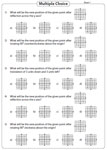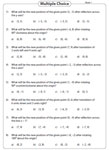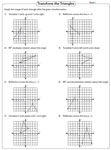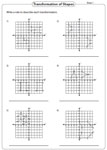1. Worksheets>
2. Math>
3. Geometry>
4. Transformation

Transformation Worksheets: Translation, Reflection and Rotation

Transformation worksheets contain skills on slides, flips, turns, translation, reflection and rotation of points and shapes. In addition, writing the coordinates of the transformed shapes and more are included.

Slide, Flip and Turn Worksheets

In these worksheets identify slides, flips and turns of the given figures. Also move the shapes as illustrated and graph them.

Translation Worksheets

Translation worksheets have ample skills to translate points and other shapes, writing the coordinates of the translated shapes and MCQs based on it.

Rotation Worksheets

Rotate points and shapes in clockwise and counterclockwise direction and graph them in these rotation worksheets.

Reflection Worksheets

Reflect the given points and the figures across the x-axis, y-axis and also across the line x = a and y = b, where a and b are parameter.

Rotation, Reflection and Translation - Mixed Review

A mixed review of problems on the concepts of translation, reflection and rotation with exercises to identify the type of transformation, transformation of shapes, writing the coordinates of the transformed shapes and more are included in these worksheets.

Printing Help - Please do not print transformation worksheets directly from the browser. Kindly download them and print.

Identify the transformation

In these worksheets identify the image which best describes the transformation (translation, reflection or rotation) of the given figure.Write the type of transformation

Each grid has the figure and the image obtained after transformation. Write, in each case the type of transformation undergone.Transformation of points: Multiple choices

Rotate, reflect and translate each point following the given rules. Choose the correct image of the transformed point.Multiple choices: Transformation

The coordinates of a point are given. Perform the required transformation and check mark the correct choice.Transformation of shapes

Translate, reflect or rotate the shapes and draw the transformed image on the grid. Each worksheet has eight problems.Transformation of triangles

Draw the transformed image of each triangle. The type of transformation to be performed is described above each question.Translate each quadrilateral and graph the image on the grid. Label the quadrilateral after transformation.Transformation: Any two of three

Two types of transformation have been performed to each figure. Choose the correct transformations undergone.Write the rules

Identify the transformation undergone by the figure and write a rule to describe each of them.Writing coordinates: With graph

Perform the required transformation for each figure and graph it. Also write the coordinates of the image obtained.Writing new coordinates

The coordinates of the figure are given. Write down the coordinates of the vertices of the image after transformation.